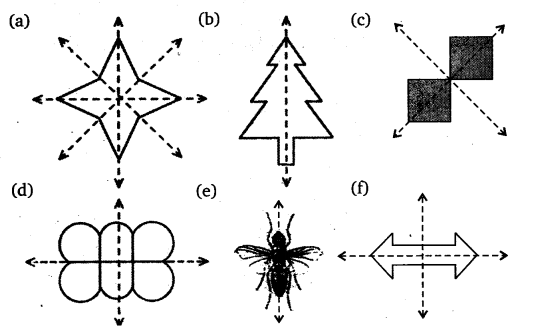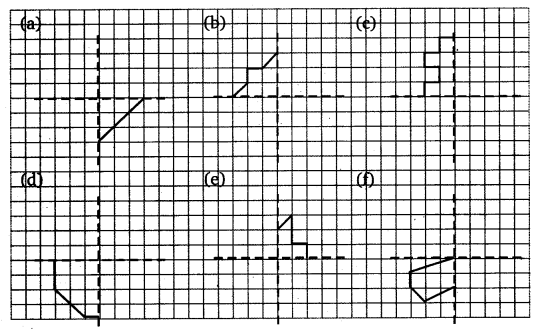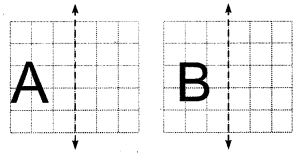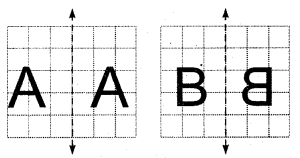# Class 6 Maths NCERT Solutions for Chapter 13 Symmetry Ex – 13.3

## Symmetry

Question 1.
Find the number of lines of symmetry in each of the following shapes: .Solution:
By drawing the line(s) of symmetry, we find that the number of line(s) possessed by them areQuestion 2.
Copy the following drawing on squared paper. Complete each one of them such that the resulting figure has two dotted lines as two lines of symmetry:How did you go about completing the picture?

Solution:
Completing the figures using the given line(s) of symmetry. The completed figures are as under:Question 3.
In each figure alongside, a letter of the alphabet is shown along with a vertical line. Take the mirror image of the letter in the given line. Find which letters look the same after reflection (i.e. which letters look the same in the image) and which do not. Can you guess why?Try for O E M N P H L T S V X

Solution:
Taking the mirror image of the letters A and B in the given line. These will look as shown.Clearly, A after reflection looks same but B does not.
It is due to the reason that the shape is preserved but sense is not.
Out of the given letters:
O, M, H, T, V, and X look as before after reflection, whereas E, P, N, L, and S does not.

#### Class 6 Maths NCERT Solutions for Chapter 14 Practical Geometry Ex – 14.1

Lorem ipsum dolor sit amet, consectetur adipiscing elit. Phasellus cursus rutrum est nec suscipit. Ut et ultrices nisi. Vivamus id nisl ligula. Nulla sed iaculis ipsum.

Company Name